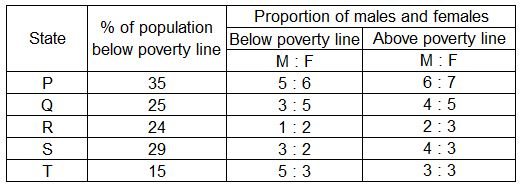## SBI PO Quant Test 14

Instructions

Study the table carefully and answer the given questions.Question 1

If the male population above poverty line for State R is 2.38 lakhs then what is the total population of R?

Question 2

What will be the number of females above poverty line in the State S if it is known that the population of State S is 7 million ?

Question 3

What is the male population above poverty line for State P if the female population below poverty line for State P is 2.1 million ?

Question 4

If the population of males below poverty line for State Q is 2.4 million and that for State T is 6 million, then what is the respective ratio of the total population of states Q and T ?

Instructions

For the following questions answer them individually

Question 5

In a village, three people contested for the post of Village Pradhan. Due to their own interest, all the voters voted and no one vote was invalid. The losing candidate got 30% votes. What could be the minimum absolute margin of votes by which the winning candidate led by the nearest rival, if each candidate got an integral percent of votes ?